# How to☝️ Use the INDIRECT Function in Google Sheets

To use the INDIRECT function in Google Sheets, select a cell, go to the Formula bar, type the formula =INDIRECT(“A1”), and hit Enter

## How to Quickly Use the INDIRECT Function in Google Sheets

Below you will find step-by-step instructions as to how the INDIRECT function works:

1. Click on the blank cell where you want the result.
2. Go to the Formula bar and enter the formula =INDIRECT(“A1”), where A1 is the cell with your value.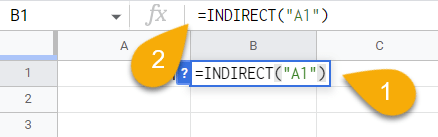3. Finally, press the Enter key on your keyboard.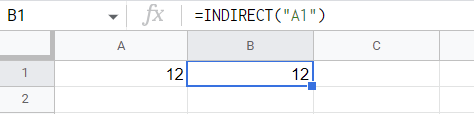And here is your result. Easy as ABC!

## Drilling Down into the INDIRECT Function

Take a look at the detailed description of the INDIRECT function below to learn all about it and how to use it on your spreadsheet.

### Description

The INDIRECT function in Google Sheets is a reference function that returns a cell or range of cells based on a text string.

For example, if you have a value in cell A1, you can apply a formula to cell B1 to reference cell A1. This is what the INDIRECT function does.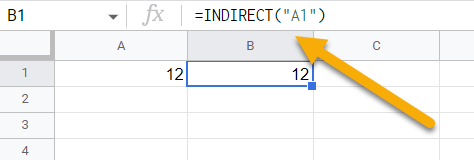### Purpose

The INDIRECT function in Google Sheets can be useful when you want to reference a cell or range of cells based on the value of another cell. You can use it to change the reference to a cell within a formula without changing the formula itself.

### Syntax

=INDIRECT(cell_reference_as_string, [is_A1_notation])

### Arguments

• сell_reference_as_string is the cell name displayed in text format.
• [is_A1_notation] is the content that explains what type of data is contained in the cell.

### Return Value

This function returns the value of a cell or range of cells based on the text string of the referenced cell.

### Usage Notes

We hope the usage notes below will be useful to you.

1. Remember to use quotation marks when entering cell names in the formula. Otherwise, a warning will appear stating that there is an error.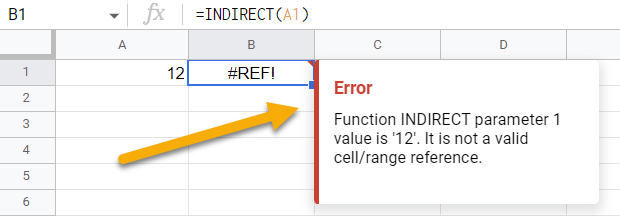2.  The INDIRECT function can be applied to a single cell or to a group of cells.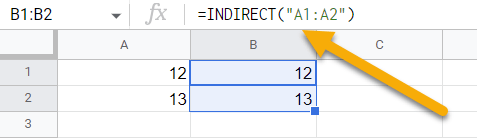3. Alternatively, you can simply use a cell reference instead of the INDIRECT function in your formula.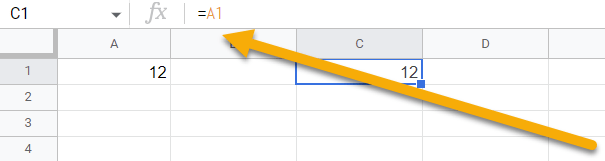### Examples

1. Using a cell name in a formula will display the contents of the cell that is being referred to in your formula:

=INDIRECT(“A1”) // Output: 12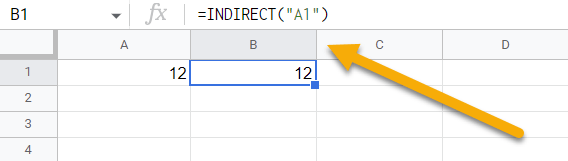1. Use only the cell reference you want:

=A1 // Output: 121. In the following formula, we entered the text value TRUE to indicate the notation A1:

=INDIRECT(“A1”, TRUE) // Output: 12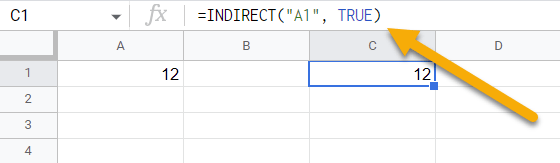1. If you refer to the row number as well as the column number instead of the cell reference, then use the formula below:

=INDIRECT(“R1C1”, False) // Output: 12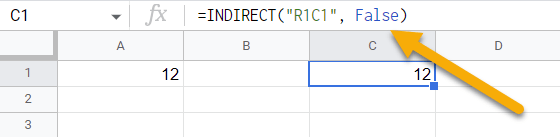1. You can get a value from another sheet using the following formula:

=INDIRECT(“Sheet1!”&”B1”) // Output: 12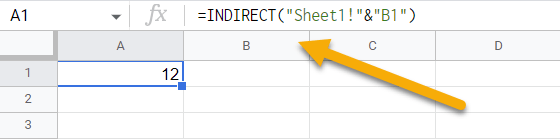1. You can also apply the INDIRECT function using a formula with sheet names:1. Use the INDIRECT function by referring to a result that contains another function.

=SUM(INDIRECT(“A1:A5”)) // Output: 70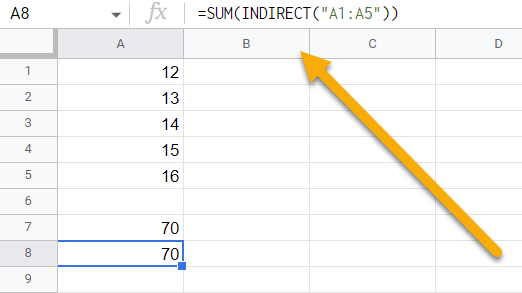## The INDIRECT Function in Google Sheets FAQs

The FAQs below will provide you with more details regarding the INDIRECT function and will help you get the most out of this function.

### When is it a good idea to avoid using INDIRECT in Google Sheets?

As the INDIRECT function is a volatile one, that means it will recalculate every time there is a change in the spreadsheet, which means it cannot be used with fixed values. This can cause slow performance and calculation errors. It is only recommended to use the INDIRECT function when necessary.

### Are there any Google Sheets formulas similar to INDIRECT?

There are no completely identical formulas for the INDIRECT function in Google Sheets. But there is one similar function that is partially similar to the INDIRECT function.

The HYPERLINK function creates a hyperlink to a cell or range of cells. It’s often used with the INDIRECT function to create dynamic links.